# Magic Stars

Magic Stars are a little like Magic Squares. My grandson was introduced to them in his junior high school math class.

### Contents

#### Family Enterprise

This post is a family enterprise. My grandson Ben heard about five-point magic stars in his junior high school math class, where they tackled them by hand. Ben told his mom Kam, my daughter, who wrote a MATLAB program, and then told me.

#### Observatory

Here is the function that I use to view the stars.

   type observatory

   function observatory(v)
% observatory  View Magic Stars.
% observatory(v), where v is a vector of 10 or 12 integers.
clf
n = length(v)/2;
d = pi/n;
t = 0:2*n-1;
r0 = .42 + .20*(n == 5);
r = 1 - r0*(mod(t,2)==1);
x = r.*sin(t*d);
y = r.*cos(t*d);
p = plot(x,y,'o','markersize',28,'markeredgecolor',[0 0 .66]);
axis(1.25*[-1 1 -1 1])
axis square
set(gca,'xtick',[],'ytick',[])
if n == 5
h = line([x(1:4:2*n) x(3:4:2*n) x(1)], ...
[y(1:4:2*n) y(3:4:2*n) y(1)]);
elseif n == 6
h = [line([x(1:4:2*n) x(1)],[y(1:4:2*n) y(1)])
line([x(3:4:2*n) x(3)],[y(3:4:2*n) y(3)])];
else
error('Can only observe 10 or 12 points')
end
set(h,'color',[0 .66 .66])
for k = 1:2*n
text(x(k),y(k),int2str(v(k)),'fontsize',18,'horiz','center')
end
end


#### Basic Stars

There are lots of kinds of magic stars. Here are the most basic. Draw a regular n-point star the way you learned to draw a 5-point star. Connect the n points evenly spaced around a circle by n lines between alternate points. This produces a regular n-gon in the interior. The vertices of the outer points, together with the vertices of the interior polygon, gives you 2n points. Here are the five and six point stars, with the points numbered in consecutive clockwise order.

   observatory(1:10)
snapnow

observatory(1:12)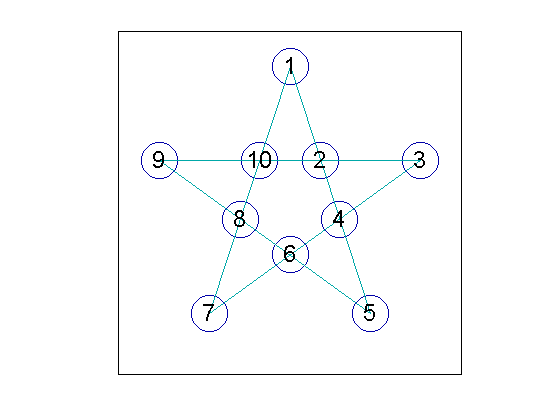#### Magic Stars

To turn one of these stars into a magic star, you need to rearrange the numbers so that the sums along each of the n lines are equal, just like the sums along the rows and columns in a magic square. It turns out that this is impossible for the 5-point star and we will have to modify the rules. But it is possible for the 6-point star.

#### Five-point

The sum of the integers from 1 to 10 is S = 55. There are five lines in the 5-point star and each integer is involved in the intersection of two of these lines, so if the sums along each line are to be equal, that sum must be L = (2/5)S = 22. We are seeking a permutation p of the first ten integers that satisfies the five equations.

$$p_1+p_2+p_4+p_5 = L$$

$$p_3+p_4+p_6+p_7 = L$$

$$p_5+p_6+p_8+p_9 = L$$

$$p_7+p_8+p_{10}+p_1 = L$$

$$p_9+p_{10}+p_2+p_3 = L$$

The subscripts in each of these equations can be read off by following one of the lines across the star.

An exhaustive search of all 10! permutations of the integers 1 through 10 reveals that there are no solutions to these equations, so no perfect five-point magic star exists.

But do not despair. Relax the rules by allowing more players. Ben's class was given the challenge of finding a magic 5-point star using nine of the ten integers between 1 and 10, and also using 12. They had to be careful. The sum S needs to be a multiple of 5 because the line sum is always going to be L = (2/5)S. So they learned that they had to exclude either 2 or 7. Let's first try leaving out the 7.

I'm going to give the integer 12 a special role by fixing it at the apex of the star. This eliminates all the stars that are obtained from the ones that I will find by simple rotations, and it also eliminates all the stars where 12 is at an internal point. It leaves only nine integers left to permute. Here are the parameters.

   clear
n = 5;
v = [1:6 8:10];   % Omit 7.
S = sum(v) + 12
L = 2/n*S

S =

60

L =

24



Use the MATLAB function perms to generate all possible permutations of the nine free integers.

   P = perms(v);


Insert a column of 12's in front to produce a matrix with 9! (that's 9 factorial) rows and 10 columns.

   P = [12*ones(size(P,1),1) P];
sizeP = size(P)

sizeP =

362880          10



See how much storage we're using.

   whos

  Name            Size               Bytes  Class     Attributes

L               1x1                    8  double
P          362880x10            29030400  double
S               1x1                    8  double
n               1x1                    8  double
sizeP           1x2                   16  double
v               1x9                   72  double



My laptop can handle 29 megabytes.

We are ready to search for the solutions.

   f = find(P(:,1)+P(:,2)+P(:,4)+P(:,5) == L & ...
P(:,3)+P(:,4)+P(:,6)+P(:,7) == L & ...
P(:,5)+P(:,6)+P(:,8)+P(:,9) == L & ...
P(:,7)+P(:,8)+P(:,10)+P(:,1) == L & ...
P(:,9)+P(:,10)+P(:,2)+P(:,3) == L)

f =

85418
99312
133775
139937
205213
222969
257655
272091
284491
298403
322934
361090



Out of the over one-third of a million permutations, these are the indices that satisfy the five line equations. Here are those solutions.

   P = P(f,:)

P =

12     8     9     1     3    10     4     6     5     2
12     8     5     3     1    10     6     4     9     2
12     6    10     4     2     9     1     8     5     3
12     6     5     2     4     9     8     1    10     3
12     4     9     2     6     5     8     3    10     1
12     4    10     6     2     5     3     8     9     1
12     3     5     8     1     9     2     4    10     6
12     3    10     1     8     9     4     2     5     6
12     2     9     4     6    10     1     3     5     8
12     2     5     6     4    10     3     1     9     8
12     1     9     8     3     5     2     6    10     4
12     1    10     3     8     5     6     2     9     4



Twelve solutions were found. The last solution has the integers from 1 to 5 in the interior.

   p = P(12,:)
observatory(p)

p =

12     1    10     3     8     5     6     2     9     4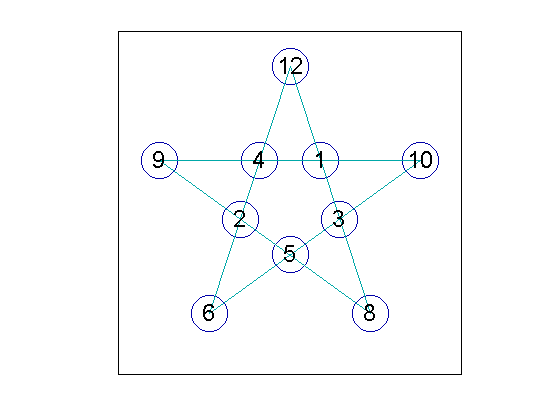#### Rotations and reflections

Our search for stars formed from the integers from 1 to 12, leaving out 7 and 11, found 12 solutions. But the last six are actually reflections of the first six. Look at the solution array P, and ignore the first column with the 12s. If you read the last six rows backwards, from right to left, you will get the first six rows in a different order. You can also think of this as clockwise versus counter clockwise indexing.

So, we have found six distinctly different solutions with 12 at the apex. There are three kinds of transformations that can be made on these solutions. This will produce all of the possible magic stars that can be made from this set of integers.

   p = p([1 10:-1:2])
observatory(p)
snapnow

p =

12     4     9     2     6     5     8     3    10     1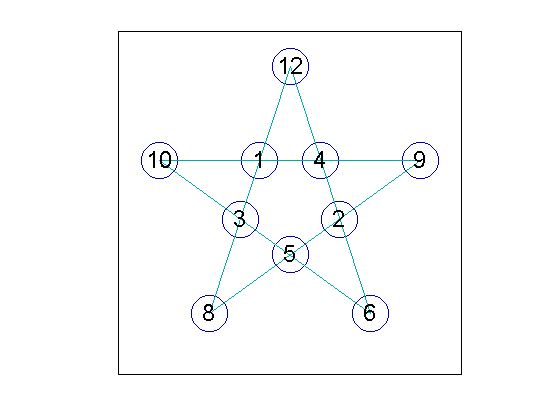Rotate, counter clockwise.

   p = p([3:10 1:2])
observatory(p)
snapnow

p =

9     2     6     5     8     3    10     1    12     4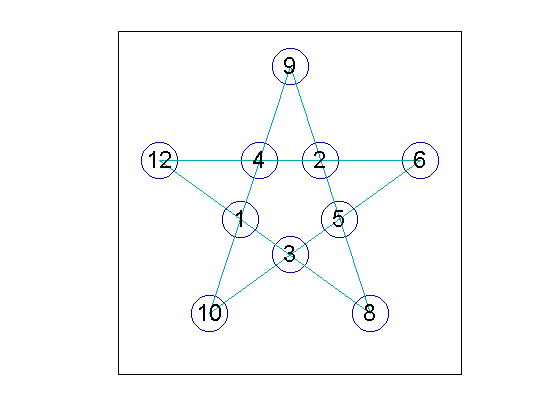Invert, interchange interior with exterior.

   p = p([6:10 1:5])
observatory(p)
snapnow

p =

3    10     1    12     4     9     2     6     5     8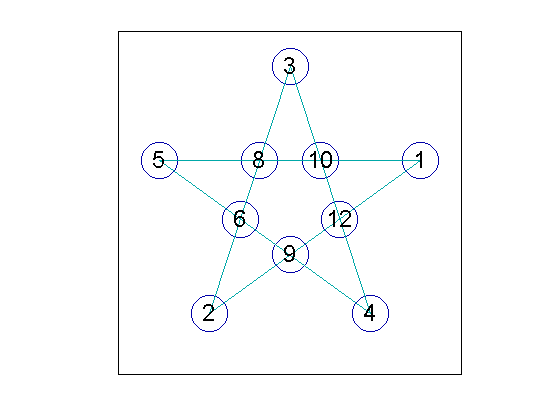Applying two reflections, five rotations, and two inversions to each of the original six solutions produces a grand total of 6*2*5*2 = 120 different magic stars.

#### More five-point stars

You can modify my code to leave out the 2 instead of the 7. Use v = [1 3:10] instead of v = [1:6 8:10]. You will find that the search for solutions comes up empty. Magic stars formed from the integers 1 through 12 without the 2 and 11 do not exist.

You can also try including 11, but leaving out 2 and 6. Use v = [1 3:5 7:11] instead of v = [1:6 8:10]. Is there an isomorphism between these stars and we ones I described above?

#### Six-point

We can make a 6-point star using the integers from 1 to 12, so we don't have to bend the rules. But I am going to restrict the search space and be more careful about memory usage.

Here is the line sum.

   clear
n = 6;
S = sum(1:2*n);
L = 2/n*S

L =

26



I have decided to restrict the search space to stars with 11 at the apex and 12 at the base. So I need to find all permutations of the remaining integers from 1 to 10. This would use ten times as much memory as I needed to do the 5-point star and my laptop can't handle it. But instead of storing the integers as the MATLAB default eight-byte double precision numbers, I can use one-byte uint8s and reduce the memory requirement by a factor of eight.

Find all permutations of ten integers, stored in single bytes.

   v = uint8(1:10);
P = perms(v);


Insert a column of 11s and a column of 12s in the appropriate places.

   c = ones(size(P,1),1,'uint8');
P = [11*c P(:,1:5) 12*c P(:,6:10)];
clear c


The matrix has 10! rows, which is about 3.6 million.

   sizeP = size(P)

sizeP =

3628800          12



But the storage required is not much more than above.

   whos

  Name             Size               Bytes  Class     Attributes

L                1x1                    8  double
P          3628800x12            43545600  uint8
S                1x1                    8  double
n                1x1                    8  double
sizeP            1x2                   16  double
v                1x10                  10  uint8



There are six line equations to be satisfied.

   f = find(P(:,1)+P(:,2)+P(:,4)+P(:,5) == L & ...
P(:,3)+P(:,4)+P(:,6)+P(:,7) == L & ...
P(:,5)+P(:,6)+P(:,8)+P(:,9) == L & ...
P(:,7)+P(:,8)+P(:,10)+P(:,11) == L & ...
P(:,9)+P(:,10)+P(:,12)+P(:,1) == L & ...
P(:,11)+P(:,12)+P(:,2)+P(:,3) == L)

f =

235278
520708
641908
1321733
1522350
1930425
2012865
2613900



Here are the eight solutions found in the 3.6 million rows. Again, the last four are reversals of the first four.

   P = P(f,:)

P =

11   10    4    2    3    8   12    6    9    1    7    5
11    9    6    1    5    7   12    4   10    2    8    3
11    9    3    1    5   10   12    4    7    2    8    6
11    7    4    2    6    8   12    3    9    1   10    5
11    6    8    2    7    4   12   10    5    1    3    9
11    5    7    1    9    6   12    8    3    2    4   10
11    5   10    1    9    3   12    8    6    2    4    7
11    3    8    2   10    4   12    7    5    1    6    9



I think the first one is quite attractive. I have not investigated all the possible symmetries and transformations.

   observatory(P(1,:))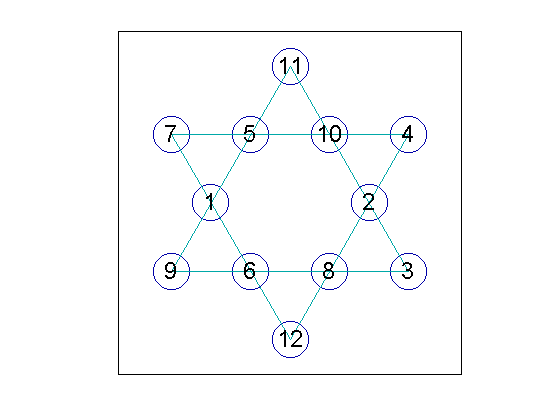Marian Trenkler, Magic Stars, PME Journal 11, 2004, 549-554

Harvey Heinz, Magic Stars Index Page, <http://www.magic-squares.net/magic_stars_index.htm>

Published with MATLAB® R2014a

|